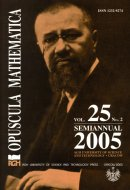Opuscula Math. 25, no. 2 (2005), 351-354

Opuscula Mathematica

# A note on self-complementary hypergraphs

Małgorzata Zwonek

Abstract. In the paper we describe all self-complementary hypergraphs. It turns out that such hypergraphs exist if and only if the number of vertices of the hypergraph is of the form $$n=2^k$$. This answers a conjecture posed by A. Szymański (see [Szymański A.: Note on self-complementary 4-uniform hypergraphs.(Preprint)]).

Keywords: self-complementary hypergraphs, complementing permutation.

Mathematics Subject Classification: 05C65.

Full text (pdf)

• Małgorzata Zwonek
• AGH University of Science and Technology, Faculty of Applied Mathematics, al. Mickiewicza 30, 30-059 Kraków, Poland